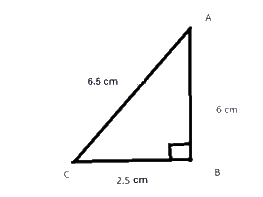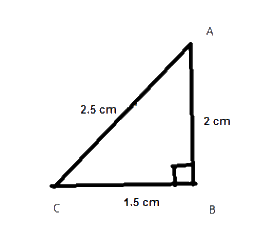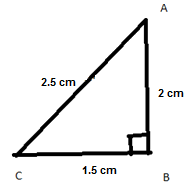# Ex.6.5 Q4 The Triangle and Its Properties - NCERT Maths Class 7

Go back to  'Ex.6.5'

## Question

Which of the following can be the sides of a right triangle?

(i) $$\rm{}2.5\, cm, \rm{}6.5\,cm, \rm{}6\,cm$$

(ii) $$\rm{}2\,cm, \rm{}2\, cm, \rm{}5\, cm.$$

(iii) $$\rm{}1.5\,cm, \rm{}2\,cm, \rm{}2.5\, cm.$$

In the case of right-angled triangles, identify the right angles.

Video Solution
Triangle & Its Properties
Ex 6.5 | Question 4

## Text Solution

What is  known?

Sides of the triangles.

What is unknown?

Given sides are the sides of right-angled triangle or not.

Reasoning:

This question is very simple. Consider the greater side as the hypotenuse and if the square of hypotenuse is equal to the sum of square of other two sides then these are the sides of a right - angled triangle.

Steps

(i)\begin{align}&{{\left( \text{Hypotenuse} \right)}^2} \\&= \rm{{ }}{{\left(\text {Perpendicular} \right)}^2} + {\rm{ }}{{\left( \text{Base} \right)}^2} \end{align}

\begin{align}{{\left( {6.5} \right)}^2} &= \rm{{ }}{{\left( 6 \right)}^2} + {{\left( {2.5} \right)}^2}\\42.25{\rm{ }} &= \rm{{ }}36 + {\rm{ }}6.25\\42.25{\rm{ }} &= \rm{{ }}42.25\\ L.H.S &= R.H.S\end{align}

Therefore, given sides are of the right-angled triangle.

Right angle lies opposite to the greater side.

(ii)\begin{align}&{{\left( \text{Hypotenuse} \right)}^2} \\&= \rm{{ }}{{\left(\text {Perpendicular} \right)}^2} + {\rm{ }}{{\left( \text{Base} \right)}^2} \end{align}

\begin{align} {{\left( 5 \right)}^2} &= \rm{{ }}{{\left( 2 \right)}^2} + {\rm{ }}{{\left( 2 \right)}^2}\\25{\rm{ }} &= \rm{{ }}4{\rm{ }} + {\rm{ }}4\\25{\rm{ }}& \ne {\rm{ }}8\end{align}

Therefore, the given sides are not of a right- angled triangle.

(iii)\begin{align}&{{\left( \text{Hypotenuse} \right)}^2} \\&= \rm{{ }}{{\left(\text {Perpendicular} \right)}^2} + {\rm{ }}{{\left( \text{Base} \right)}^2} \end{align}

\begin{align} {{\left( {2.5} \right)}^2} &= \rm{{ }}{{\left( 2 \right)}^2} + {\rm{ }}{{\left( {1.5} \right)}^2}\\6.25{\rm{ }} &= \rm{{ }}4{\rm{ }} + {\rm{ }}2.25\\6.25{\rm{ }} &= \rm{{ }}6.25\\L.H.S&= R.H.S\end{align}

Therefore, given sides are of a right-angled triangle. Right angle lies on the opposite to the
greater side.

Useful Tip:

Whenever you encounter problem of this kind, it is best to think of the Pythagoras property
that if the square of hypotenuse or greater side is equal to the sum of square of other two sides then it is a right- angled triangle.

Learn from the best math teachers and top your exams

• Live one on one classroom and doubt clearing
• Practice worksheets in and after class for conceptual clarity
• Personalized curriculum to keep up with school